• ## R语言条形图

千次阅读 2018-04-09 09:14:05
R语言使用函数barplot()创建条形图R语言可以在条形图中绘制垂直和水平条。 在条形图中，每个条可以给予不同的颜色。 语法 在R语言中创建条形图的基本语法是 - barplot(H, xlab, ylab, main, names.arg, ...
条形图表示矩形条中的数据，条的长度与变量的值成比例。 R语言使用函数barplot()创建条形图。 R语言可以在条形图中绘制垂直和水平条。 在条形图中，每个条可以给予不同的颜色。
语法
在R语言中创建条形图的基本语法是 -
barplot(H, xlab, ylab, main, names.arg, col)
以下是所使用的参数的描述 -
H是包含在条形图中使用的数值的向量或矩阵。xlab是x轴的标签。ylab是y轴的标签。main是条形图的标题。names.arg是在每个条下出现的名称的向量。col用于向图中的条形提供颜色。
例
使用输入向量和每个条的名称创建一个简单的条形图。  以下脚本将创建并保存当前R语言工作目录中的条形图。
# Create the data for the chart.
H <- c(7,12,28,3,41)

# Give the chart file a name.
png(file = "barchart.png")

# Plot the bar chart.
barplot(H)

# Save the file.
dev.off()
当我们执行上面的代码，它产生以下结果 -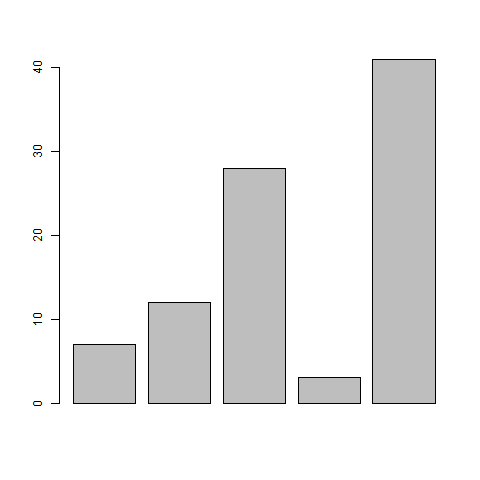条形图标签，标题和颜色
可以通过添加更多参数来扩展条形图的功能。 主要参数用于添加标题。 col参数用于向条形添加颜色。 args.name是具有与输入向量相同数量的值的向量，以描述每个条的含义。
例
以下脚本将在当前R语言工作目录中创建并保存条形图。
# Create the data for the chart.
H <- c(7,12,28,3,41)
M <- c("Mar","Apr","May","Jun","Jul")

# Give the chart file a name.
png(file = "barchart_months_revenue.png")

# Plot the bar chart.
barplot(H,names.arg = M,xlab = "Month",ylab = "Revenue",col = "blue",
main = "Revenue chart",border = "red")

# Save the file.
dev.off()
当我们执行上面的代码，它产生以下结果 -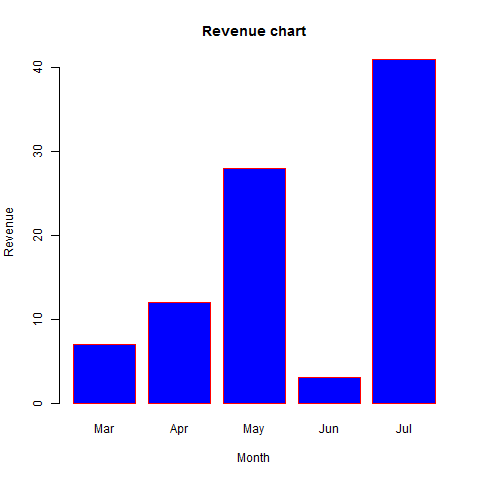组合条形图和堆积条形图
我们可以使用矩阵作为输入值，在每个条中创建条形图和堆叠组的条形图。  超过两个变量表示为用于创建组合条形图和堆叠条形图的矩阵。
# Create the input vectors.
colors <- c("green","orange","brown")
months <- c("Mar","Apr","May","Jun","Jul")
regions <- c("East","West","North")

# Create the matrix of the values.
Values <- matrix(c(2,9,3,11,9,4,8,7,3,12,5,2,8,10,11),nrow = 3,ncol = 5,byrow = TRUE)

# Give the chart file a name.
png(file = "barchart_stacked.png")

# Create the bar chart.
barplot(Values,main = "total revenue",names.arg = months,xlab = "month",ylab = "revenue",
col = colors)

# Add the legend to the chart.
legend("topleft", regions, cex = 1.3, fill = colors)

# Save the file.
dev.off()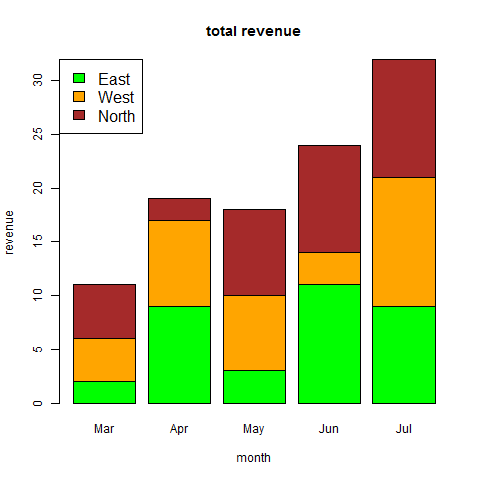展开全文•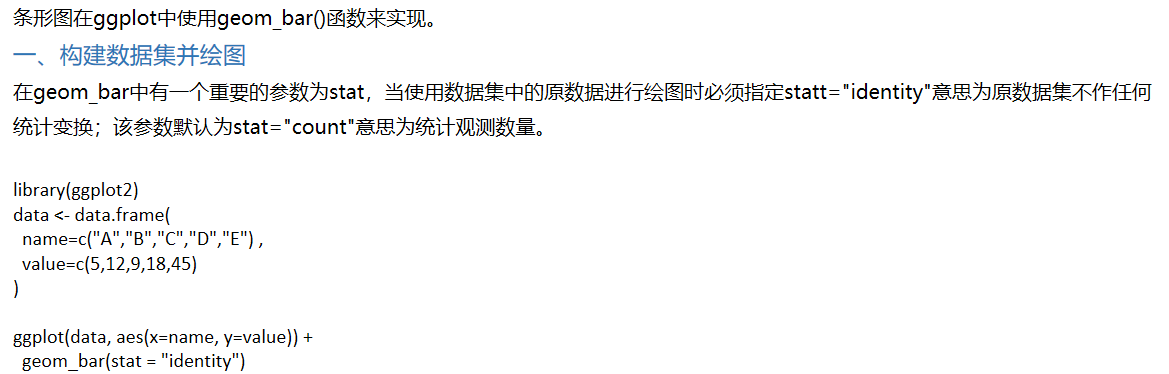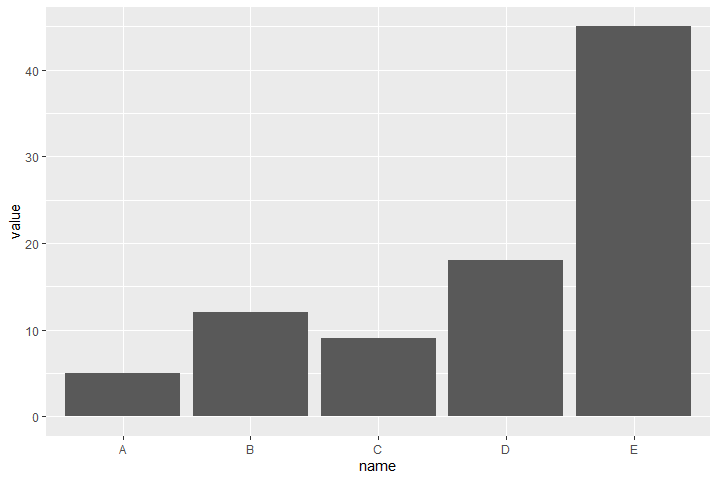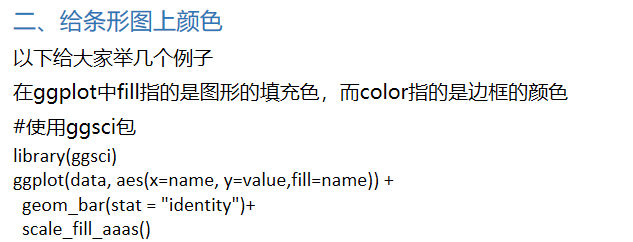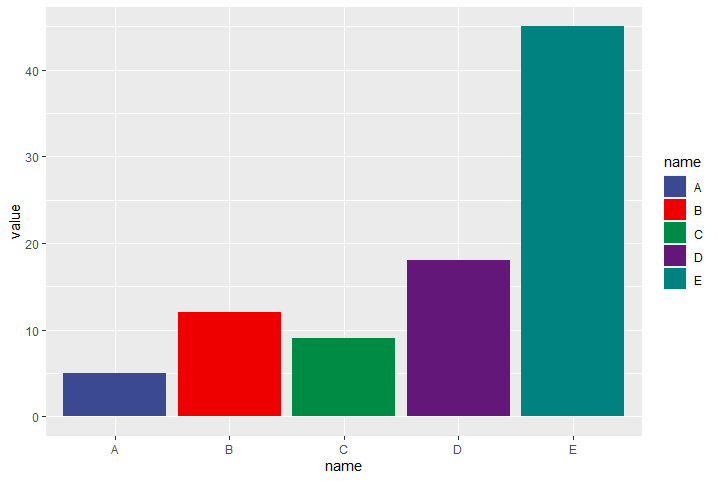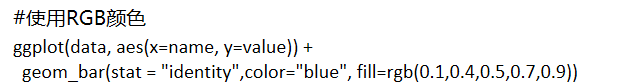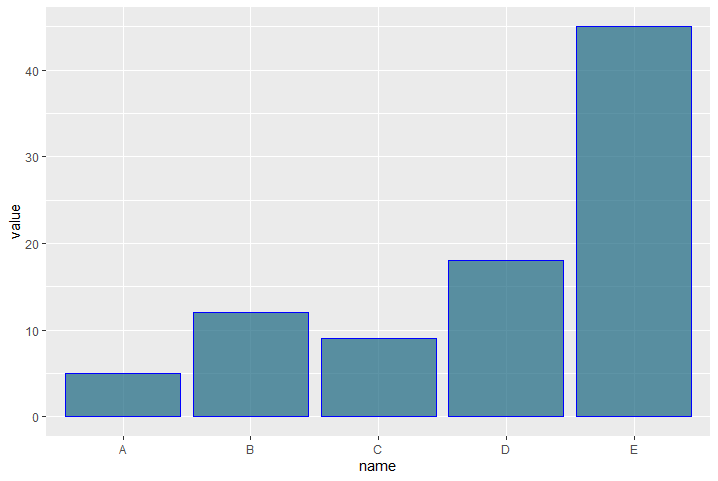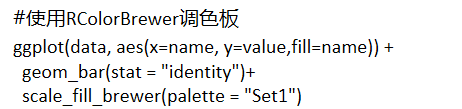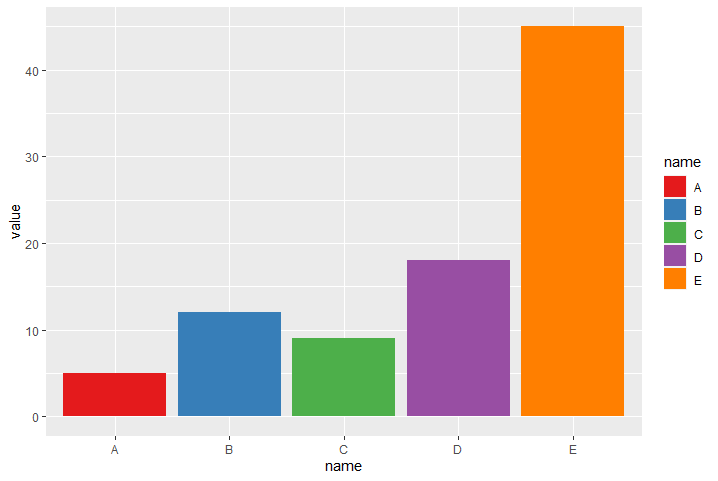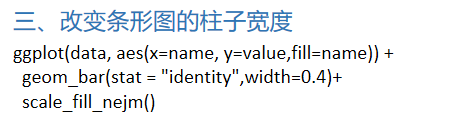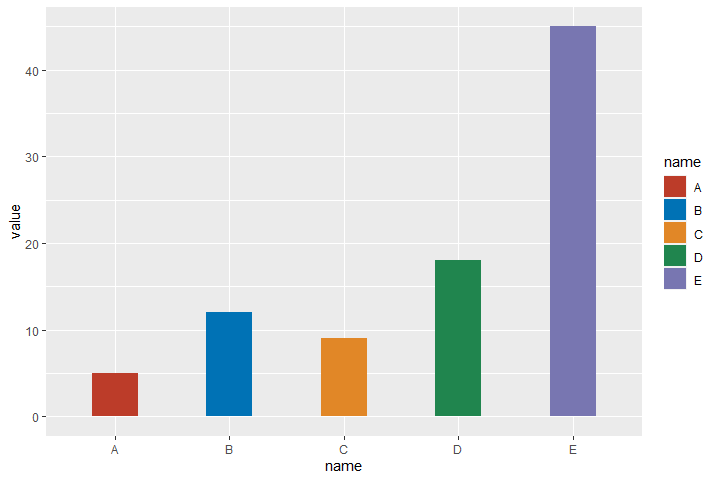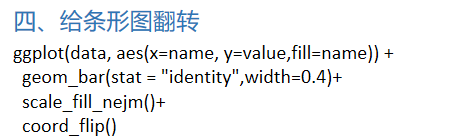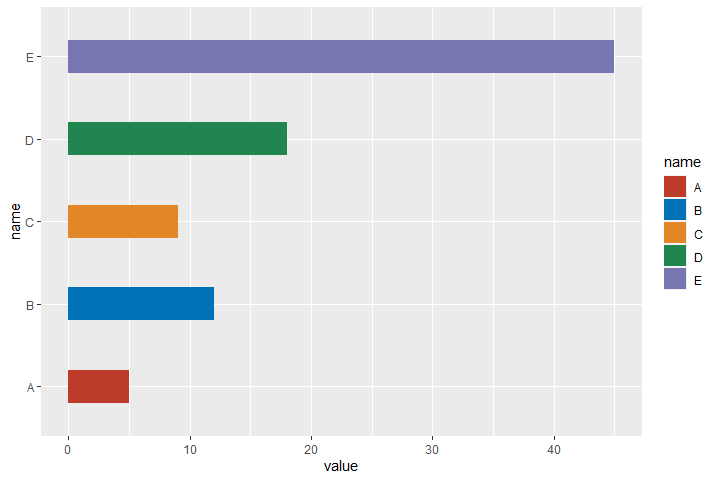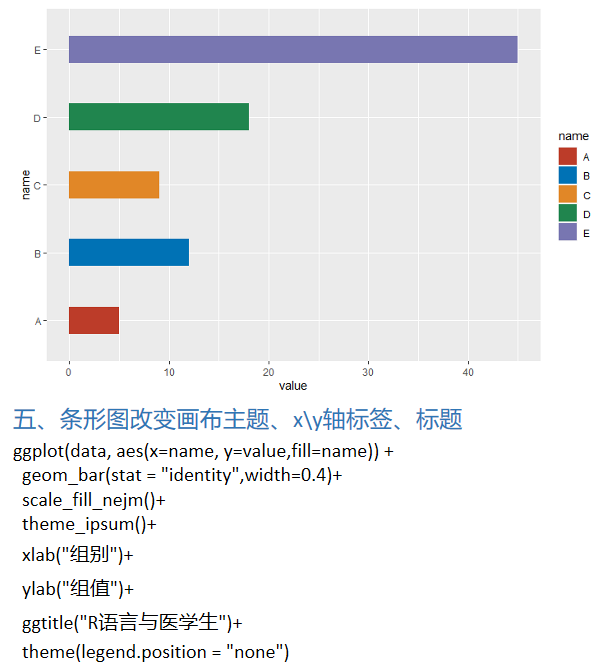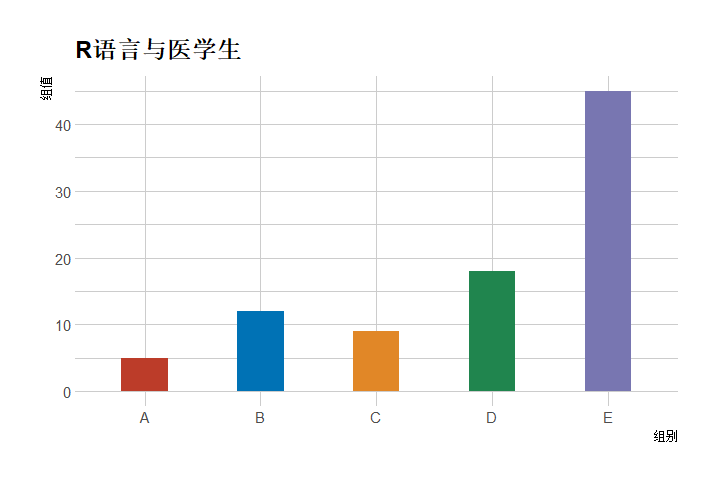展开全文• 条形图通过条形的高度或者长短来...R语言的base包里面有barplot可以绘制条形图。ggplot2包里面有geom_bar可以绘制。 barplot barplot函数 barplot(height, width = 1, space = NULL, names.arg = NULL, legend.te...
条形图通过条形的高度或者长短来表示数据多少的图形。用来展示分类变量（categorical）的组成和分布。 R语言的base包里面有barplot可以绘制条形图。ggplot2包里面有geom_bar可以绘制。
barplot
barplot函数
barplot(height, width = 1, space = NULL,
names.arg = NULL, legend.text = NULL, beside = FALSE,
horiz = FALSE, density = NULL, angle = 45,
col = NULL, border = par("fg"),
main = NULL, sub = NULL, xlab = NULL, ylab = NULL,
xlim = NULL, ylim = NULL, xpd = TRUE, log = "",
axes = TRUE, axisnames = TRUE,
cex.axis = par("cex.axis"), cex.names = par("cex.axis"),
inside = TRUE, plot = TRUE, axis.lty = 0, offset = 0,
add = FALSE, ann = !add && par("ann"), args.legend = NULL, ...)

horiz，默认是FALSE, 条形图是垂直的。设置为TRUE后，条形图会变成水平的。 subset，在有多个属性的data.frame数据上，subset会用到。选出一部分数据作画。 beside，默认是FALSE, height变量会在不同的分类变量下展现为堆砌条形图。设置为TRUE,会变为并列条形图。
简单条形图
例子1
> studentGender <- c("Female","Male")
> studentNumber <- c(340, 234)
> barplot(studentNumber~studentGender )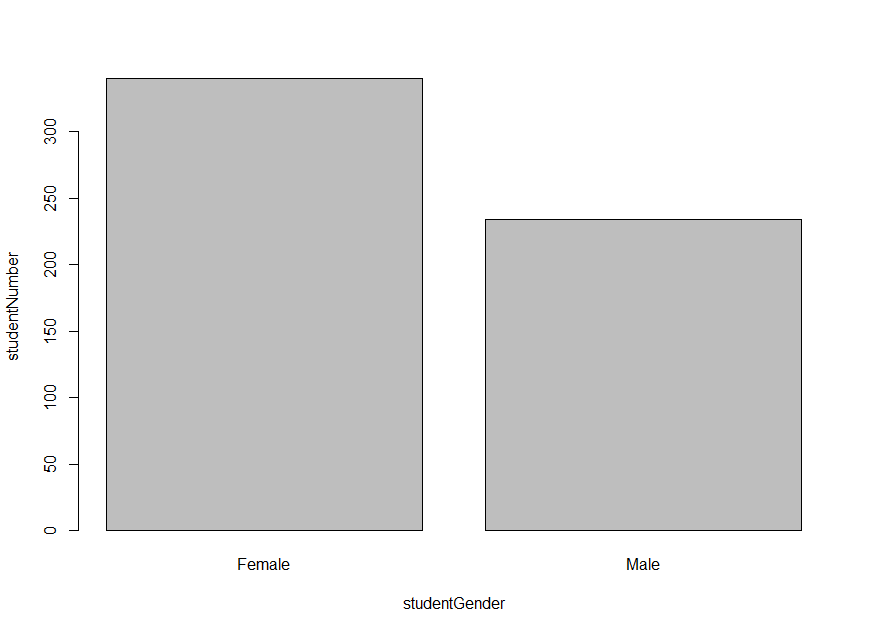例子2
我们画图用到的数据是data.frame的Titanic.
summary(d.Titanic <- as.data.frame(Titanic))
Class       Sex        Age     Survived      Freq
1st :8   Male  :16   Child:16   No :16   Min.   :  0.00
2nd :8   Female:16   Adult:16   Yes:16   1st Qu.:  0.75
3rd :8                                   Median : 13.50
Crew:8                                   Mean   : 68.78
3rd Qu.: 77.00
Max.   :670.00

Class    Sex   Age Survived Freq
1   1st   Male Child       No    0
2   2nd   Male Child       No    0
3   3rd   Male Child       No   35
4  Crew   Male Child       No    0
5   1st Female Child       No    0
6   2nd Female Child       No    0

barplot(Freq ~ Class, data = d.Titanic, subset = Age == "Adult" & Sex == "Male" & Survived=="Yes")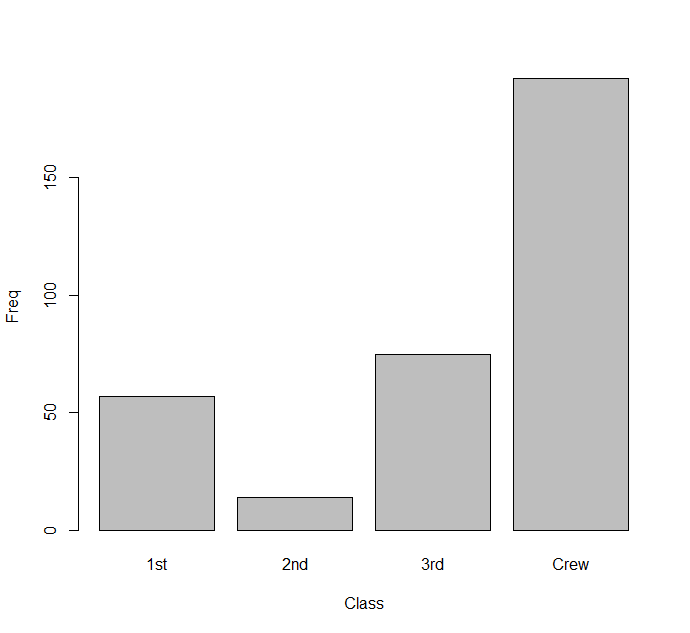堆砌条形图 stacked bars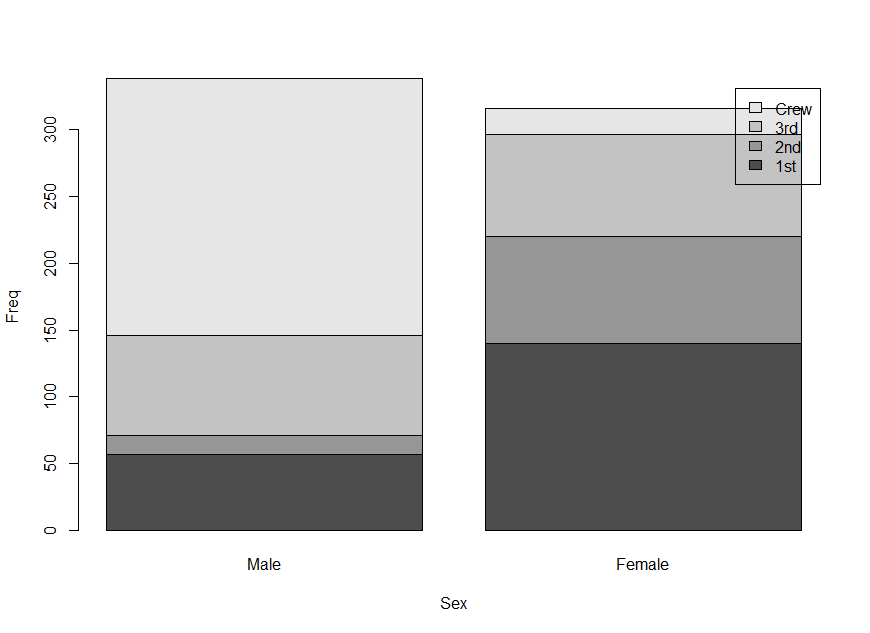barplot(Freq ~ Class + Sex, data = d.Titanic, subset = Age == "Adult" & Survived=="Yes", legend = TRUE)

分组条形图 group bars
group bars又叫 juxtaposed bars。默认的barplot在多个分布属性下生成的是stacked bars。将函数参数beside设置为TRUE，就是分组条形图。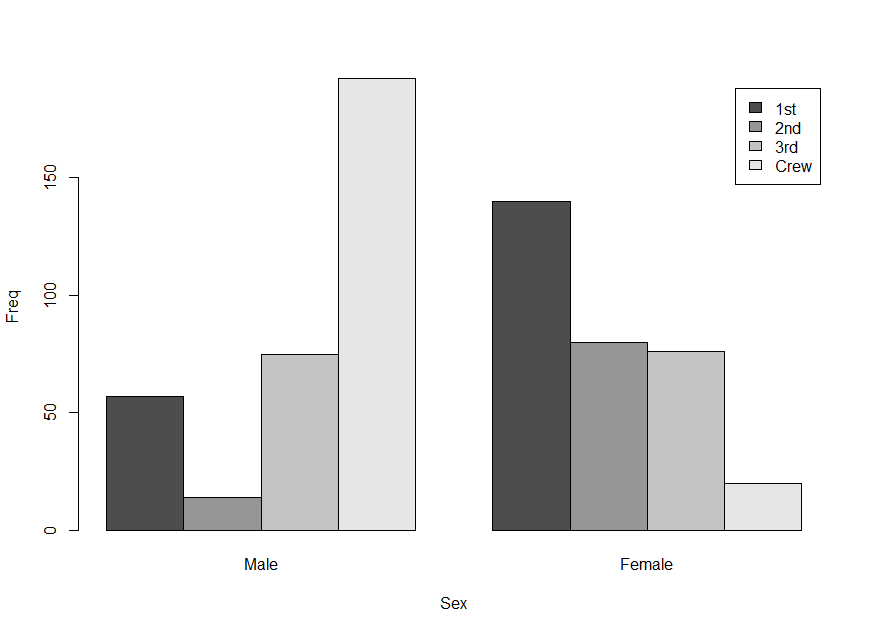barplot(Freq ~ Class + Sex, data = d.Titanic, subset = Age == "Adult" & Survived=="Yes", beside=TRUE, legend = TRUE)

条形图设置颜色
在基于上面分组条形图的基础上，设置了颜色。用到了包RColorBrewer.
library(RColorBrewer)
mypalette<-brewer.pal(4,"Greens")
summary(d.Titanic <- as.data.frame(Titanic))
barplot(Freq ~ Class + Sex, data = d.Titanic, subset = Age == "Adult" & Survived=="Yes", beside=TRUE, legend = TRUE, col=mypalette)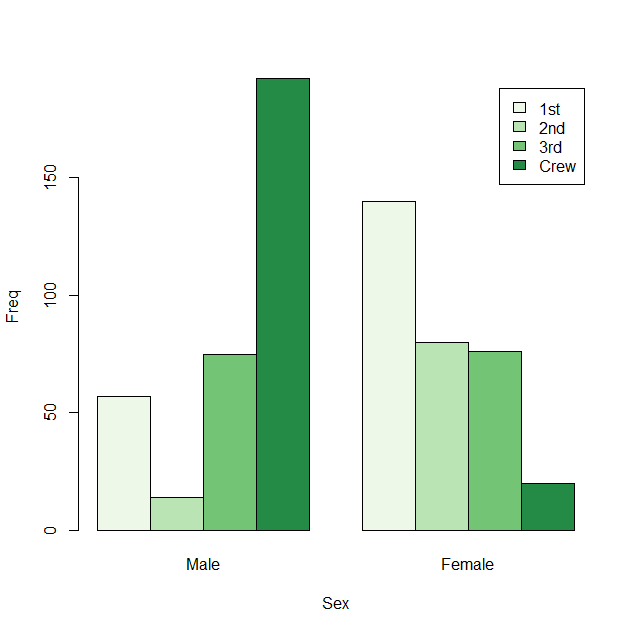ggplot2 条形图
简单条形图
library("ggplot2")
studentGender <- c("Female","Male")
studentNumber <- c(340, 234)
studentData <- data.frame(studentGender, studentNumber)
ggplot(studentData, aes(studentGender, studentNumber)) +
geom_bar(stat="identity")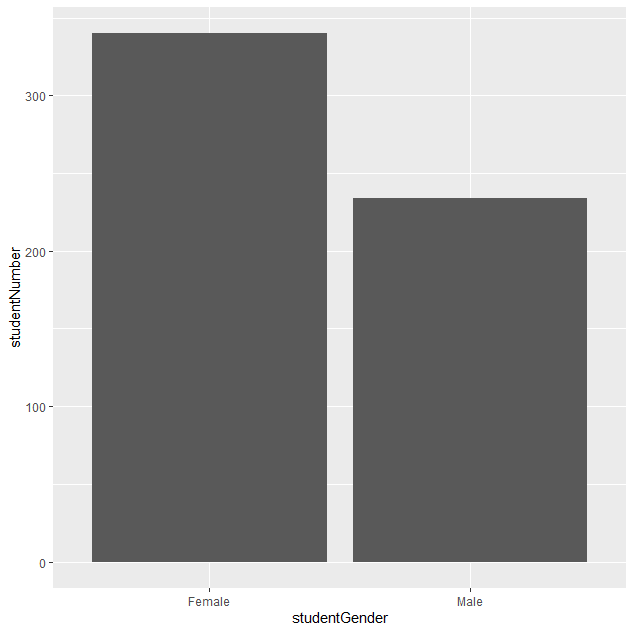堆砌条形图 stacked bars
library("ggplot2")
summary(d.Titanic <- as.data.frame(Titanic))
ggplot(d.Titanic , aes(Class, Freq)) +
geom_bar(stat="identity",aes(fill=Sex))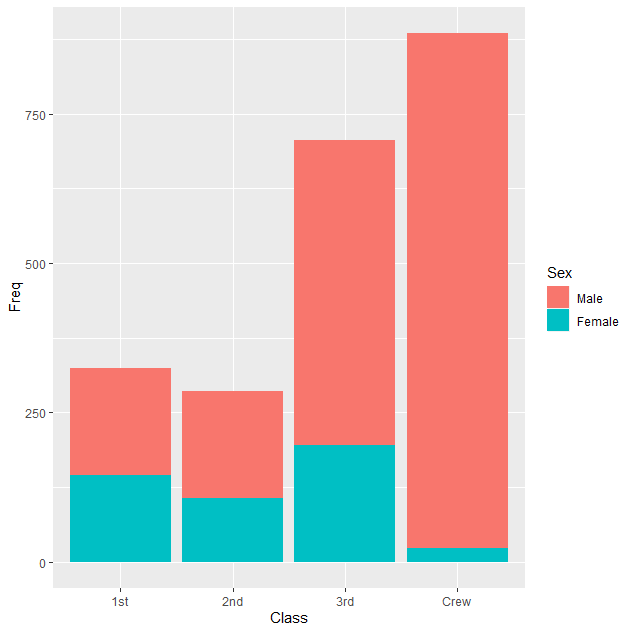分组条形图 group bars
基于上面的R script, 设置geom_bar的position参数为dodge，会生成分组条形图。
summary(d.Titanic <- as.data.frame(Titanic))
ggplot(d.Titanic , aes(Class, Freq)) +
geom_bar(stat="identity",position="dodge",aes(fill=Sex))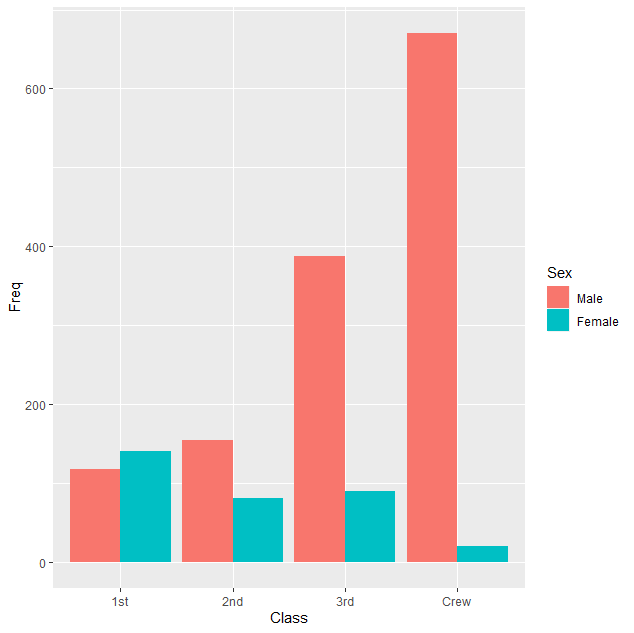我们可以在上面的script加上coord_flip将图形反转一下。
summary(d.Titanic <- as.data.frame(Titanic))
ggplot(d.Titanic , aes(Class, Freq)) +
geom_bar(stat="identity",aes(fill=Sex)) +
coord_flip()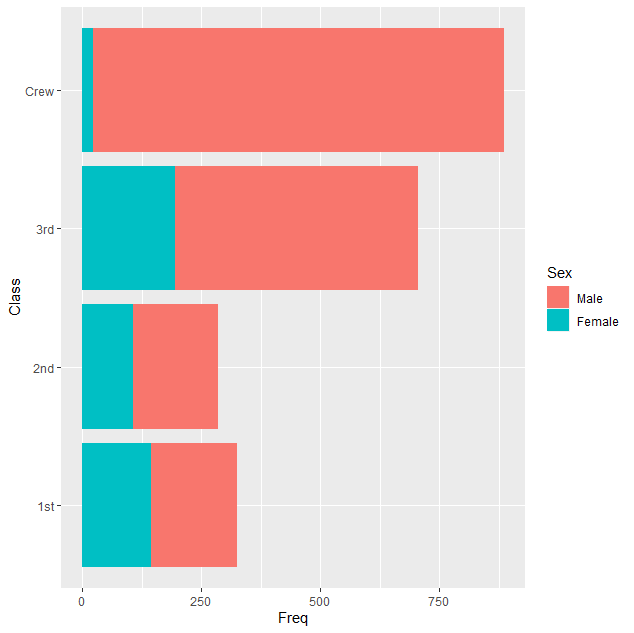展开全文• 函数barplot()可以绘制条形图，其格式为 barplot(height) height是一个向量或者矩阵，使用horiz=TRUE可以生成一个水平的条形图， 例子 1，绘制简单的条形图 注意一般条形图都是统计分类变量每一钟元素的频数，此时...
函数barplot()可以绘制条形图，其格式为 barplot(height) height是一个向量或者矩阵，使用horiz=TRUE可以生成一个水平的条形图， 例子 1，用条形图统计分类变量的频数 注意条形图常用来统计分类变量每一钟元素的频数，此时可以运用table()进行处理分类变量，其可以统计分类变量的各个元素的频次。处理后的结果为table格式而barplot()可以识别table格式 table()函数可以统计列各种元素出现的次数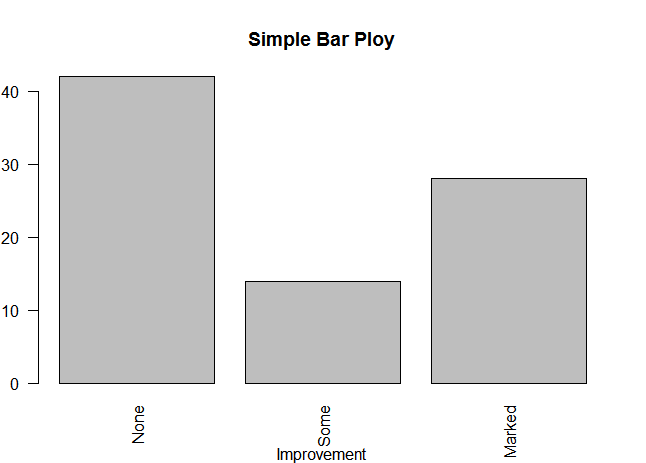counts <- table(Arthritis$Improved) counts barplot(counts,xlab = "Improvement",ylab = "Frequency",main = "Simple Bar Ploy")  例子2 生成水平的条形图 barplot()参数horiz可以指定图形是否为水平的条形图 注意生成的水平的条形图时，x,y轴的名称不会跟着变换所以x,y轴的名称应同时跟着变换 barplot(counts,horiz = TRUE ,ylab = "Improvement",xlab = "Frequency",main = "Simple Bar Ploy")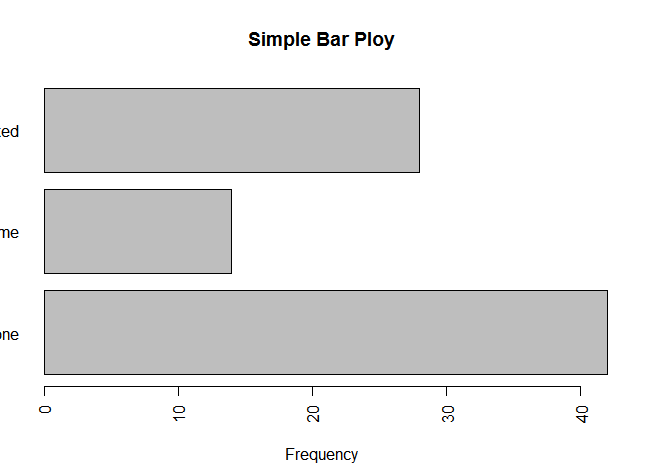例子3，使用plot()函数绘制条形图 如果像上面的例子那样，绘制的类别型变量是一个因子或有序因子，可以直接使用plot()函数快速创建条形图，这样就不用再通过table()函数进行处理变量。图片效果与例子1相同 plot(Arthritis$Improved,xlab = "Improvement",ylab = "Frequency",main = "Simple Bar Ploy")
#绘制水平的条形图
plot(Arthritis$Improved,horiz=TRUE,xlab = "Improvement",ylab = "Frequency",main = "Simple Bar Ploy")  例子3绘制堆砌条形图和分组条形图 如果height是一个矩阵而不是一个向量，则绘制结果将是一幅堆砌条形图或分组条形图 当beside=FALSE(默认值），则生成堆砌条形图–矩阵的每一列都将生成一个条形 当beside=TRUE，则生成分组条形图–矩阵中的每一列都表示一个分组，各列的中值将并列而不是堆砌 注意barplot()中的height要么是向量要么是矩阵，不能是data.frame但是可以是table格式 opar <- par(no.readonly = TRUE) par(opar) par(mfrow=c(1,2)) counts <- table(Arthritis$Improved,Arthritis$Treatment) counts #绘制堆砌条形图 barplot(counts,main = "Stacked Bar Plot",xlab = "Treatment",ylab="Frequency", col = c("red","yellow","green"),legend=row.names(counts)) #绘制分组条形图 barplot(counts,beside = TRUE,main = "Stacked Bar Plot",xlab = "Treatment",ylab="Frequency", col = c("red","yellow","green"),legend=row.names(counts))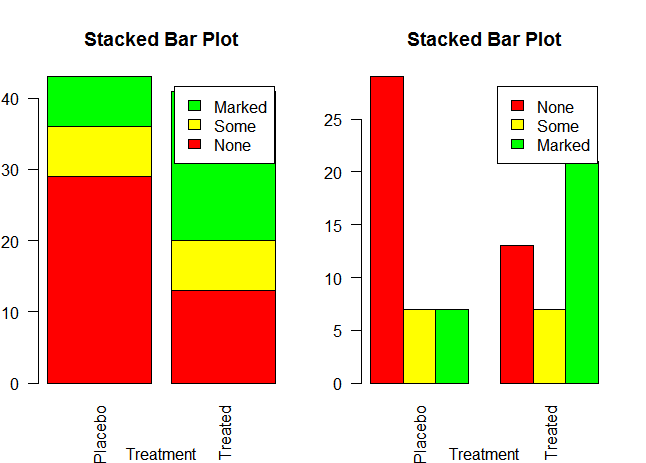例子4，使用数据整合函数 aggregate()并将结果传递给barplot()函数，来创建表示均值，中位数，标准值等条形图 能够如此作图的原因是因为我们可以向作图函数barplot()只传递一个数值向量，再通过参数names.arg指定横坐标的标签，（arg为英文自变量的含义） 这样我们我们输入各个分组后各组的平均值的值向量、在通过参数names.arg指定各组名称为横坐标标签就可以制作成一个条形图了 #使用数据整合函数aggregate()并将结果传递给barplot()函数，来创建表示均值，中位数，标准差等条形图 #生成要作图的数据框 states <- data.frame(state.region,state.x77) #根据列state.region对列states$Illiteracy进行分组并求每组的均值。
#by = list(state.region)指定分组变量
#states$Illiteracy为被分组的变量 #FUN=mean是指定处理被分组的变量的函数 means <- aggregate(states$Illiteracy,by = list(state.region),FUN=mean)
#设定数值只能保留2位小数
options(digits = 2)
#barplot()函数可以输入没有索引的向量，但是这个时候就没有横坐标的标签，
#这时就需要通过names.arg参数指定（arg为自变量的意思）
par(opar)
barplot(means$x,names.arg = means$Group.1)
title("Mean Illiteracy Rate")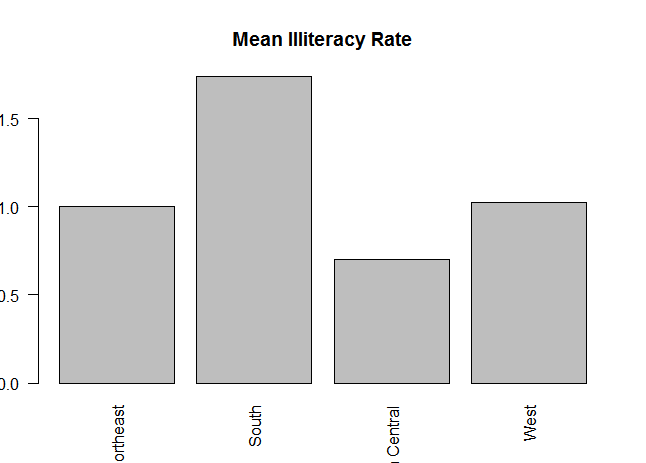例子5，条形图的微调 cex.names参数调整字号 names.arg指定字符向量作为条形的标签图 las可以调整标签的参数，las=2为水平方向,las=0为垂直方向
par(mar=c(5,8,4,2))
#las调整标签的参数，2为水平，0为垂直
par(las=2)
library(vcd)
counts <- table(Arthritis\$Improved)
barplot(counts,
main = "Treatment Outcome",
horiz = TRUE,
#调整标签字体大小
cex.names = 0.8,
#修改标签文本
names.arg = c("No Improvement","Some Improvement","Marked Improvement"))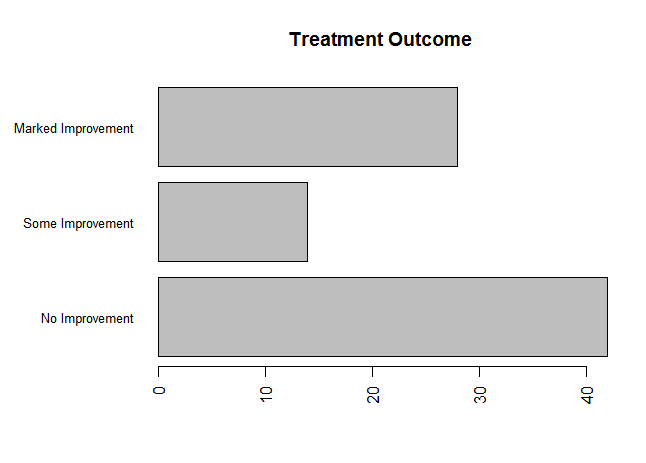例子6，棘状图 棘状图–棘状图对堆砌条形图进行了重缩放，这样让每列生成的条形图的高度均为1，换句话说它把每一列的数值替换为此数值在这一列数值总和的占比。 棘状图可以由vcd包中的函数spine()绘制
library(vcd)
attach(Arthritis)
counts <- table(Treatment,Improved)
spine(counts,main="Spinogram Example")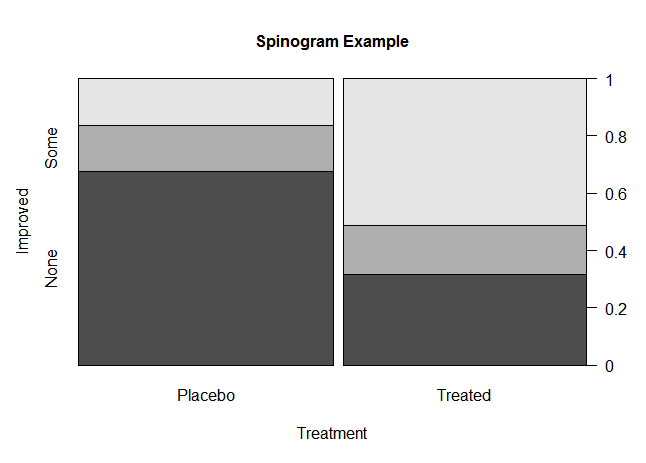结束总结 能够制作条形图的函数有三个分别是barplot()函数，plot()函数和vcd包中的spine()函数 plot()函数可以直接输入分类变量，不用再通过table()处理分类变量把它变成含有分类变量各个元素频数的表格。 spine()函数可以生成棘状图 条形图可以绘制成水平的，但是此时应注意修改x轴坐标的名称 当需要绘制条形图的是一个数据框时此时会生成堆砌或者分组的条形图。生成什么样的条形图由参数beside决定，当beside=FALSE时生成堆砌的条形图（每一列的数值将堆砌在一个条形上），否则生成分组的条形图 当函数barplot(）的参数height是一个数值向量时（只有数值不附带索引），此时可以通过names.arg参数指定x轴刻度的标签
展开全文• 数据结果的图形展示，R代码，《R数据科学》是个好东西 数据格式如下： term category pval neutrophil chemotaxis biological_process 1.68E-09 innate immune response biological_process ...
• 环状条形图非常适合于周期性数据，本文主要介绍基于R语言实现环状条形图的绘制。本文主要参考链接:Circular barplot R语言的环状条形图主要基于tidyverse包实现，tidyverse是一组R包的集合，这些R包共享共同的原理并...
• 制作简单条形图 在500到1000间创建5个均匀分布的随机数,利用names()函数将x进行命名,再将"Group"1到5做成横坐标,最后利用barplot()函数创建条形图
• R语言对于统计图的绘制可以说是非常有效了，本文使用ggplot2包里的qplot函数绘制条形图。大家一快学习使用。那个对ggplot2语法不了解的同学也可以前来参观。因为该案例不会涉及太对的语法。 1、数据简介 本文的数据...
• barplot（height）是一个向量或者矩阵，即简单条形图和堆积条形图，默认是绘制垂直条形图。 beside=FALSE:堆砌条形图 beside=TRUE：分组条形图 表示均值，中位数、标准差的条形图：使用数据整合函数来生成条形图。 ...数据可视化 可视化
• 在使用堆积条形图时候，新增一个百分比堆积条形图，可以加深读者印象。 封装一个function函数后只需要在调用的数据上改一下pos=‘fill’的代码即可。比较方便。 案例： # 封装函数 fun1<-function(data,...
• 网购次数和满意度的条形图。其结果如下所示 (1) 使用 read.csv()读取数据到 data3_1 中，可以采用绝对路径或者相对路 径形式读取文件。 (2) 在 R 控制台查看 data3_1 的内容 (3) 查看 data3_1 的模式 (4) 使用 ...
• R语言条形图barplot 条形图通过垂直的或水平的条形展示了类别型变量的分布（频数）。函数 barplot() 函数的格式为：barplot(height, width = 1, space = NULL, names.arg = NULL, legend.text = NULL, beside = ...
• 条形图表示矩形条中的数据，其长度与变量的值成比例，R使用barplot()函数来创建条形图R可以在条形图中绘制垂直和水平条，并且，在条形图中，每个条可以被赋予不同的颜色，语法如下： barplot(H, xlab, ylab, ...
• 1.绘制基础的条形图； # 加载包 library(ggplot2) # 模拟数据集 data <- data.frame( name=c("Q","W","E","G","E","H","J","K","L","M") , value=c(2,14,3,17,50,68,71,64,32,99) ) # 绘图 ggplot(data, aes...
• 基本分组条形图2. 使柱子并排放置3. 改变条形图配色4. 使纵坐标呈现百分比5. 删掉图例名称 1. 基本分组条形图 假设我们有如下数据，其大致结构如图。 dat = data.frame(专业=rep(c('统计','计算机'),each = 2), ...ggplot2 统计学 数据可视化 数据分析
• 分享一波环状条形图的代码，可以自己动手试试~ library(tidyverse) # 创建数据集 data <- data.frame( individual=paste( "Mister ", seq(1,60), sep=""), group=c( rep('A', 10), rep('B', 30), rep('C', 14)...
• 簇状条形图的绘制与美化建立数据绘制簇状条形图条形图着色条的大小更改条形图间间距堆积条形图完整代码仅供参考创作不易，都浏览到这儿了，看官可否将下面的收藏点赞帮忙点亮。 建立数据 巧妇难为无米之炊，按例我得...可视化 数据可视化...